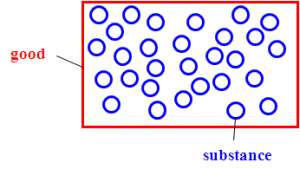## System of Equations

In order to describe the model in a mathematical way 3 types of equations are used:

1. Mass conservation
e.g. balance equation
sum of inputs = sum of outputs + change in stockor
e.g. stock equation
stock i = stock i -1+ change in stock i-1
with i…period

i.e. the amount of stock at the beginning of a period can be calculated from the amount of stock in the previous period plus the change in stock during this previous period.
2. Linear relations
e.g. transfer coefficient equation
output 1 = TC1 * Sum of inputs
output 2 = TC2 * Sum of inputs
with TC1 + TC2 = 1or
e.g. additional linear relation equation between similar quantities
flow x = factor * flow y
3. Concentration equation
mass substance = mass good * concentration substanceor
mass substance = volume good * concentration substance
mass good = volume good * density good